#### 2018 02 01The riskyr data structures essentially describe networks of dependencies. This is best illustrated by the network diagram (see examples of plot_fnet in the user guide and data formats). However, sometimes it is instructive to view all possible values of some parameter as a function of others. A functional perspective illustrates how the value of some parameter (or its values) changes as a function of other parameters (and their values).

## Functions

The basic format of a function is $$y = f(x)$$, which illustrates how values of $$y$$ depend on values of $$x$$ given some function $$f$$. riskyr provides 2 functions for viewing parameters as a function of other parameters (and their values).

## Curves as a function of prevalence

The function plot_curve draws the curves (or lines) of selected parameters as a function of the prevalene (with prev ranging from 0 to 1) for a given decision process or diagnostic test (i.e., given values of sens and spec):

$y \ = \ f(\texttt{prev} \textrm{, from 0 to 1}) \textrm{ with } y \in \{\texttt{PPV}, \texttt{NPV}, \texttt{ppod}, \texttt{acc}\} \ \ \ \ \ \ (1)$

As an example, reconsider our original scenario (on mammography screening, see user guide). Earlier, we computed a positive predictive value (PPV) of 7.8%. But rather than just computing a single value, we could ask: How do values of PPV develop as a function of prevalence? The plot_curve function illustrates this relationship:

plot_curve(prev = .01, sens = .80, spec = (1 - .096),
what = c("prev", "PPV", "NPV"),
title.lbl = "Mammography screening", cex.lbl = .8)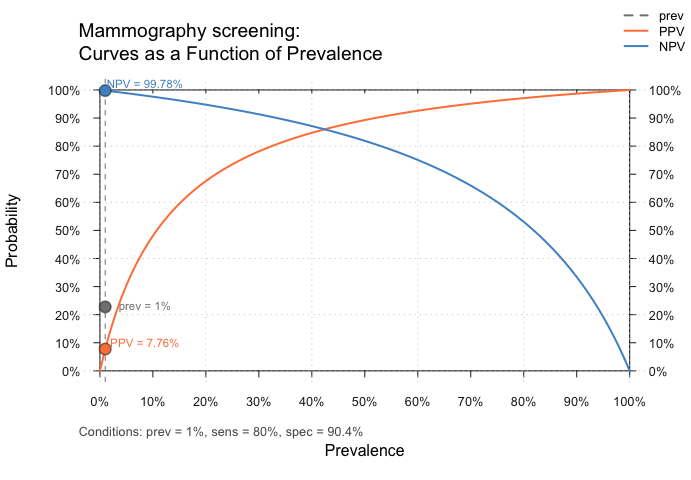Curves that show PPV and NPV as a function of an prevalence (for given values of sensitivity and specificity) in the original mammography screening scenario.

The curves illustrate that values of PPV and NPV crucially depend on the value of prevalence prev in the current population. In fact, they actually vary across their entire range (i.e., from 0 to 1), rendering any communication of their value utterly meaningless without specifying the current population’s prevalence value prev.

The dependency of PPV and NPV on prev can be illustrated by assuming a higher prevalence rate. For instance, if we knew that some woman was genetically tested and known to exhibit the notorious BRCA1 mutation, the prevalence value of her corresponding population (given a positive mammography result in a routine screening) is increased to about 60%:

high.prev <- .60   # assume increased prevalence due to BRCA1 mutation

plot_curve(prev = high.prev, sens = .80, spec = (1 - .096),
what = c("prev", "PPV", "NPV"),
title.lbl = "Mammography screening (BRCA1 mutation)", cex.lbl = .8)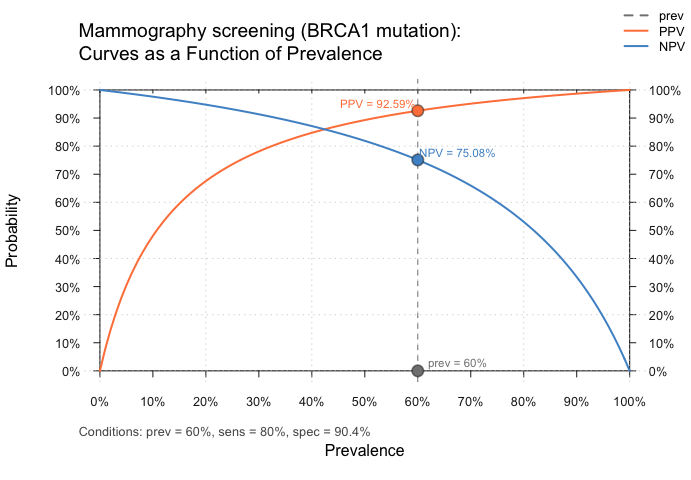Curves that show PPV and NPV as a function of an prevalence (for given values of sensitivity and specificity) when assuming an increased prevalence of 60%.

This shows that — given an increased prevalence value prev of 60% — the positive predictive value PPV of a positive test result increases from 7.8% (in the standard population) to around 93% (given the BRCA1 mutation).

Other curves (or rather lines) drawn by plot_curve include the proportion of positive decisions ppod and overall accuracy acc, each as a function of prevalence prev:

high.prev <- .60   # assume increased prevalence due to BRCA1 mutation

plot_curve(prev = high.prev, sens = .80, spec = (1 - .096),
what = c("prev", "PPV", "NPV", "ppod", "acc"),
title.lbl = "Mammography screening (BRCA1 mutation)", cex.lbl = .8)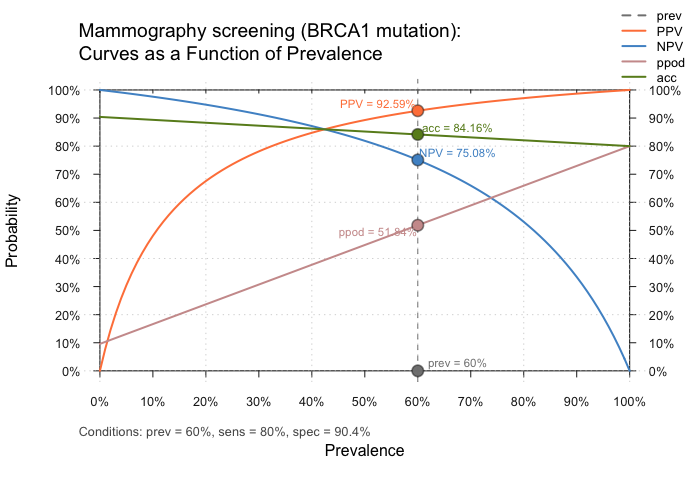Curves that show PPV and NPV as a function of an prevalence (for given values of sensitivity and specificity) when assuming an increased prevalence of 60%.

## Planes as a function of sensitivity and specificity (for given prevalence)

The function plot_plane draws a plane for a selected parameter as a function of sensitivity and specificity (with sens and spec both ranging from 0 to 1) for a given prevalence prev:

$y \ = \ f(\texttt{sens} \times\ \texttt{spec} \textrm{, both from 0 to 1, for given value of } \texttt{prev}) \textrm{ with } y \in \{\texttt{PPV}, \texttt{NPV}, \texttt{ppod}, \texttt{acc}\} \ \ \ \ \ \ \ (2)$

Some examples:

plot_plane(prev = high.prev, sens = .80, spec = (1 - .096), what = "PPV",  title.lbl = "A. Mammography (BRCA1)", cex.lbl = .8)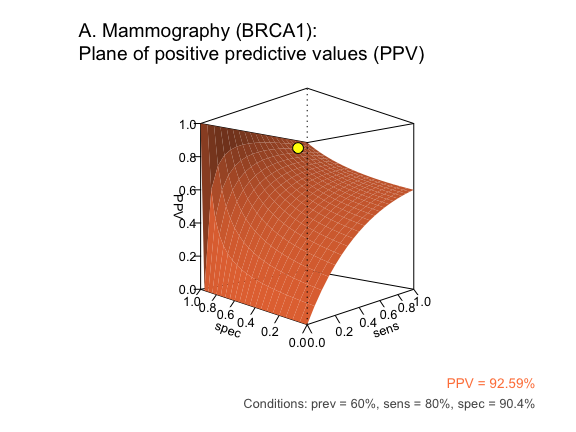Planes that show PPV, NPV, the proportion of positive predictions (ppod) and overall accuracy (acc) as a function of sensitivity and specificity (for given prevalence).

plot_plane(prev = high.prev, sens = .80, spec = (1 - .096), what = "NPV",  title.lbl = "B. Mammography (BRCA1)", cex.lbl = .8)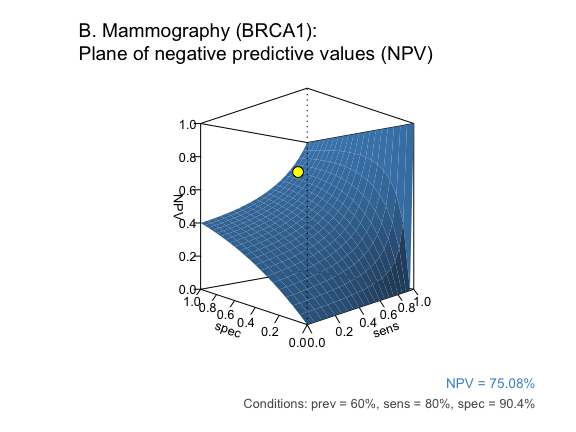Planes that show PPV, NPV, the proportion of positive predictions (ppod) and overall accuracy (acc) as a function of sensitivity and specificity (for given prevalence).

plot_plane(prev = high.prev, sens = .80, spec = (1 - .096), what = "ppod", title.lbl = "C. Mammography (BRCA1)", phi = 45, cex.lbl = .8)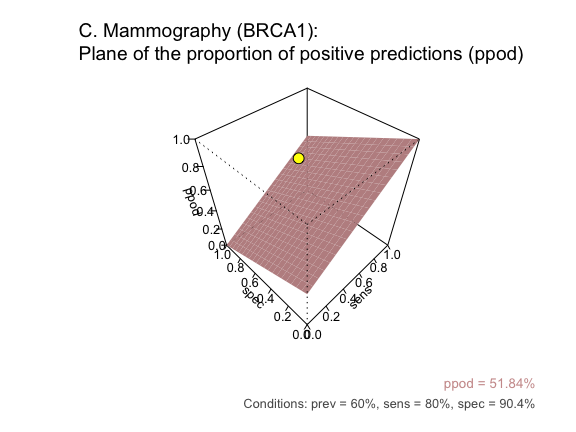Planes that show PPV, NPV, the proportion of positive predictions (ppod) and overall accuracy (acc) as a function of sensitivity and specificity (for given prevalence).

plot_plane(prev = high.prev, sens = .80, spec = (1 - .096), what = "acc",  title.lbl = "D. Mammography (BRCA1)", cex.lbl = .8)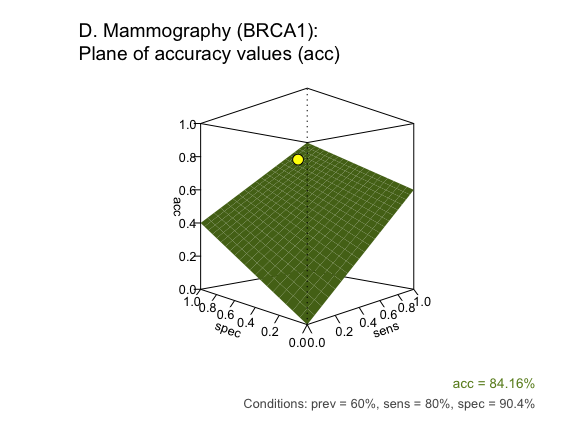Planes that show PPV, NPV, the proportion of positive predictions (ppod) and overall accuracy (acc) as a function of sensitivity and specificity (for given prevalence).